Strong solution

(diff) ← Older revision | Latest revision (diff) | Newer revision → (diff)

of a differential equation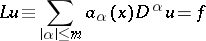in a domainA locally integrable functionthat has locally integrable generalized derivatives of all orders(cf. Generalized derivative), and satisfies

almost-everywhere in.

The notion of a "strong solution" can also be introduced as follows. A functionis called a strong solution of

if there are sequences of smooth (for example,) functions,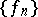such that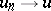,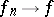and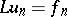for each, where the convergence is taken in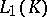for any compact set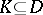. In these definitions,can be replaced by the class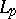of functions whose-th powers are locally integrable. The class most often used is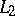.

In the case of an elliptic equation

both notions of a strong solution coincide.